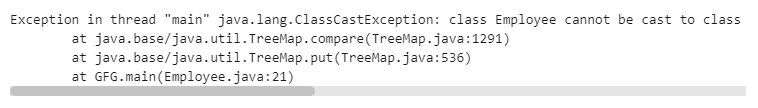Related Articles

# How to Create TreeMap Objects using Comparable Interface in Java?

• Last Updated : 21 Sep, 2021

Comparable interface is found in java.lang package which is used to order the objects of a user-defined class on the basis of a single attribute only. This interface contains a single method compareTo(Object). It is used to compare the current object with the specified objects and can be used o sort user-defined classes, wrapper class, and string objects.

Syntax:

`compareTo(Object o) ;`

Parameter: The specified object with which the current object is being compared.

Return Type: Integer value

• Positive integer — If the current object is greater than the specified object.
• Negative integer — If the current object is lesser than the specified object.
• Zero — If the current object is equal to the specified object.

Use of Comparable Interface in Java

TreeMap in Java, elements are stored as key-value pairs which are sorted on the basis of the key. When the Key is of String class or Wrapper Classes, it implements the Comparable interface by default and stores the elements in sorted order. However, if somebody wants to make the desired key of a particular user-defined type i.e user-defined class, we need to implement the Comparable interface to order the objects in a particular order on the basis of an attribute.

Implementation: Without using the comparable interface.

## Java

 `// Creating TreeMap objects using``// Comparable interface in Java` `// Importing all generic java utility and input``import` `java.io.*;``import` `java.util.*;` `// Class - User defined named Employee``public` `class` `Employee {` `    ``// Attributes of object of class``    ``int` `id;``    ``String name;` `    ``// Parameterized constructor for user-defined class``    ``public` `Employee(``int` `id, String name)``    ``{``        ``// This keyword refers to current object in a``        ``// constructor``        ``this``.id = id;``        ``this``.name = name;``    ``}``}` `// Main class``class` `GFG {` `    ``// Main driver method``    ``public` `static` `void` `main(String[] args) ``throws` `Exception``    ``{``        ``// Declaring and initializing a TreeMap``        ``TreeMap tm = ``new` `TreeMap<>();` `        ``// Employee object1``        ``// custom input``        ``Employee e1 = ``new` `Employee(``1``, ``"Pathak"``);` `        ``// Employee object2``        ``// custom input``        ``Employee e2 = ``new` `Employee(``2``, ``"Anshu"``);` `        ``// Put method associating specific key-value in Map``        ``tm.put(e1, ``"First"``);``        ``tm.put(e2, ``"Second"``);` `        ``// Iterating over Map using for-each loop``        ``// Map with employee Key``        ``for` `(Map.Entry e :``             ``tm.entrySet()) {` `            ``// Print key-value pairs of TreeMap``            ``System.out.println(e.getKey().id + ``" "``                               ``+ e.getValue());``        ``}``    ``}``}`

Output: ErrorThe above code throws exceptions as the comparable interface has not been implemented and hence it cannot order the map elements on the basis of a key in proper order. So, in order to handle the exception

Implementation: With using the comparable interface.

## Java

 `// Creating TreeMap objects using``// Comparable interface in Java` `// Importing all generic java utility and input``import` `java.io.*;``import` `java.util.*;` `// User-defined class named Employee``// implementing comparable``public` `class` `Employee ``implements` `Comparable {` `    ``// Attributes of object of class``    ``int` `id;``    ``String name;` `    ``// Parameterized constructor for user-defined class``    ``public` `Employee(``int` `id, String name)``    ``{``        ``// This keyword refers to``        ``// current object in a constructor``        ``this``.id = id;``        ``this``.name = name;``    ``}` `    ``// Comparable interface``    ``public` `int` `compareTo(Employee e)``    ``{``        ``// Two instance of class can be compared``        ``int` `diff = ``this``.id - e.id;` `        ``// Note: Two equal employee Id will return 0``        ``return` `diff;``    ``}``}` `// Main class``class` `GFG {` `    ``// Main driver method``    ``public` `static` `void` `main(String[] args)``    ``{``        ``// Declaring and initializing a TreeMap``        ``TreeMap tm = ``new` `TreeMap<>();` `        ``// Employee object1 (custom input)``        ``Employee e1 = ``new` `Employee(``1``, ``"Pathak"``);` `        ``// Employee object2 (custom input)``        ``Employee e2 = ``new` `Employee(``2``, ``"Anshu"``);` `        ``// Put method associating specific key-value in Map``        ``tm.put(e1, ``"First"``);``        ``tm.put(e2, ``"Second"``);` `        ``// Iterating over Map using for-each loop``        ``// Map with employee key``        ``for` `(Map.Entry e :``             ``tm.entrySet()) {` `            ``// Print key-value pairs of TreeMap``            ``System.out.println(e.getKey().id + ``" "``                               ``+ e.getKey().name + ``" "``                               ``+ e.getValue());``        ``}``    ``}``}`

Output
```1 Pathak First
2 Anshu Second```

Attention reader! Don’t stop learning now. Get hold of all the important Java Foundation and Collections concepts with the Fundamentals of Java and Java Collections Course at a student-friendly price and become industry ready. To complete your preparation from learning a language to DS Algo and many more,  please refer Complete Interview Preparation Course.

My Personal Notes arrow_drop_up